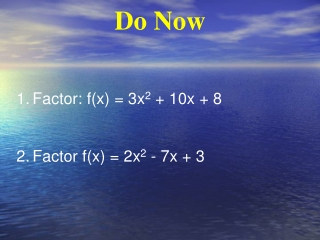DownloadDownload PresentationDo Now

# Do Now

Download Presentation## Do Now

- - - - - - - - - - - - - - - - - - - - - - - - - - - E N D - - - - - - - - - - - - - - - - - - - - - - - - - - -
##### Presentation Transcript

1. Do Now • Factor: f(x) = 3x2 + 10x + 8 • Factor f(x) = 2x2 - 7x + 3

2. Today’s Question: Today’s Question: How do you graph quadratic functions in vertex form? What important characteristics do you see in the vertex form?

3. Standard Form • A function of the form y=ax2+bx+c where a≠0 making a u-shaped graph called a parabola. Example quadratic equation:

4. Let’s Review What is the Vertex? • The lowest or highest point of a parabola. Vertex What is the Axis of Symmetry? • The vertical line through the vertex of the parabola. Axis of Symmetry

5. Vertex Form Equation y=a(x-h)2+k • If a is positive, parabola opens up If a is negative, parabola opens down. • The vertex is the point (h,k). • The axis of symmetry is the vertical line x=h. • Don’t forget about 2 points on either side of the vertex! (5 points total!)

6. Vertex Form • Every function can be written in the form (x – h)2 + k, where (h , k) is the vertex of the parabola, and x = h is its axis of symmetry. • (x – h)2 + k – vertex form

7. Example 1: Graph y = (x + 2)2 + 1 • Analyze y = (x + 2)2 + 1. • Step 1 Plot the vertex (-2 , 1) • Step 2 Draw the axis of symmetry, x = -2. • Step 3 Find and plot two points on one side , such as (-1, 2) and (0 , 5). • Step 4 Use symmetry to complete the graph, or find two points on the • left side of the vertex.

8. With a partner: Find the key characteristics: f(x) = -.5(x+3)2+4 • Does parabola open up of down? • Vertex is (h,k) • Axis of symmetry x = • Table of values x y -1 2 -2 3.5 -3 4 -4 3.5 -5 2 Vertex (-3,4) (-4,3.5) (-2,3.5) (-5,2) (-1,2) x=-3

9. Now you try one!

10. Changing from vertex or intercepts form to standard form • The key is to FOIL! (first, outside, inside, last) • Ex: y=-(x+4)(x-9) Ex: y=3(x-1)2+8 =-(x2-9x+4x-36) =3(x-1)(x-1)+8 =-(x2-5x-36) =3(x2-x-x+1)+8 y=-x2+5x+36 =3(x2-2x+1)+8 =3x2-6x+3+8 y=3x2-6x+11

11. Converting from standard to vertex fom http://www.virtualnerd.com/algebra-2/quadratics/solve-by-completing-square-roots/complete-square/completing-square-convert-standard-to-vertex

12. Challenge Problem • Write the equation of the graph in vertex form.

13. x=1 (-1,0) (3,0) (1,-8)